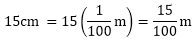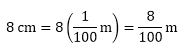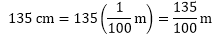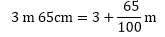×#### Thank you for registering.

One of our academic counsellors will contact you within 1 working day.

Click to Chat

1800-1023-196

+91-120-4616500

CART 0

• 0

MY CART (5)

Use Coupon: CART20 and get 20% off on all online Study Material

ITEM
DETAILS
MRP
DISCOUNT
FINAL PRICE
Total Price: Rs.

There are no items in this cart.
Continue Shopping• Complete JEE Main/Advanced Course and Test Series
• OFFERED PRICE: Rs. 15,900
• View Details

```Chapter 7: Decimals – Exercise 7.6

Question: 1

Express as rupees (Rs) using decimals

i) 15 paisa

ii) 5 paisa

iii) 350 paisa

iv) 2 rupees 60 paisa

Solution:

i)  We know that 100 paisa = Rs. 1

Therefore, 1 paisa = Rs. 1/100

15 paisa = 15/100

= Rs 0.15

ii)  We know that 100 paisa = Rs. 1

Therefore, 1 paisa = Rs. 1/100

5 paisa = 5/100

= Rs 0.05

iii) We know that 100 paisa = Rs. 1

Therefore, 1 paisa = Rs. 1/100

35 paisa = 350/100

= Rs 3.50

iv)  We know that 100 paisa = Rs. 1

Therefore, 1 paisa = Rs. 1/100

2 rupees 60 paisa = 2 + 60100

= Rs 2.60

Question: 2

Express as metres (m) using decimals

i) 15 cm

ii) 8 cm

iii) 135 cm

iv) 3 m 65 cm

Solution:

i) We know that 100 cm = 1m

Therefore 1 cm = 1/100 m0.15 m

ii)  We know that 100 cm = 1m

Therefore 1 cm = 1/100 m= 0.08 m

iii)  We know that 100 cm = 1m

Therefore 1 cm = 1/100 m= 1.35 m

iv)  We know that 100 cm = 1m

Therefore 1 cm = 1/100 m= 3.65 m

Question: 3

Express as centimetre (cm) using decimals

i) 5 mm

ii) 60 mm

iii) 175 mm

iv) 4 cm 5 mm

Solution:

i) We know that 10 mm = 1cm

Therefore 1 mm = 1/10 cm

5 mm = 5/10 cm

= 0.5 cm

ii) We know that 10 mm = 1cm

Therefore 1 mm = 1/10 cm

60 mm = 60/10 cm

= 6 cm

iii) We know that 10 mm = 1cm

Therefore 1 mm = 1/10 cm

175 mm = 175/10 cm

= 17.5 cm

iv)  We know that 10 mm = 1cm

Therefore 1 mm = 1/10 cm

4 cm 5 mm = 4 + 5/10

= 4.5 cm

Question: 4

Express as kilogram (km) using decimals

i) 5 m

ii) 55 m

iii) 555 m

iv) 5555 m

v) 15 km 35 m

Solution:

i) We know that 1000 m = 1 km

Therefore 1m = 1/1000 km

5 m = 5/1000 km

= 0.005 km

ii) We know that 1000 m = 1 km

Therefore 1m = 1/1000 km

55 m = 55/1000 km

= 0.055 km

iii) We know that 1000 m = 1 km

Therefore 1m = 1/1000 km

555 m = 555/1000 km

= 0.555 km

iv) We know that 1000 m = 1 km

Therefore 1m = 1/1000 km

5m = 5/1000 km

= 5.555 km

v) We know that 1000 m = 1 km

Therefore 1m = 1/1000 km

15 km 35 m = 15 + 351000

= 15.035 km

Question: 5

Express each of the following without using decimals

i) 8g

ii) 150 g

iii) 2750 g

iv) 5 kg 750 g

v) 36 kg 50 g

Solution:

i) We know that 1000g = 1kg

Therefore 1 g = 1/1000 kg = 0.001 kg

8g = 8/1000 kg

= 0.008 kg

ii)  We know that 1000 g = 1kg

Therefore 1g = 1/1000 kg = 0.001 kg

150 g = 150/1000 kg

= 0.150 kg

iii) We know that 1000 g = 1kg

Therefore 1 g = 1/1000 kg = 0.001 kg

2750g = 2750/1000 kg

= 2.750 kg

iv) We know that 1000 g = 1kg

Therefore 1 g = 1/1000 kg = 0.001 kg

5 kg 750 g = 5 + 7501000

= 5.750 kg

v) We know that 1000 g = 1kg

Therefore 1g =1/1000 kg = 0.001 kg

36 kg 50 g = 36 + 501000

= 36.050 kg

Question: 6

Express each of the following without using decimals

i) Rs.5.25

ii) 8.354 kg

iii) 3.05 km

iv) 7.54 m

v) 15.005 kg

vi) 12.05 m

Solution:

i) We know 100 paisa = 1 rupee

So, 1 paisa = 1/100 rupee

Therefore, Rs 5.25 = 5 + 0.25

= 5 + 25100 = Rs 5 and 25 paisa

ii) We know that 100g = 1kg

So 1 g = 1/1000 kg

Therefore, 8.354 = 8 + 0.354 = 8 + 3541000 = 8 kg 354 g

1. 3.5 cm

We know that 10 mm = 1cm

So, 1 mm = 1/10 cm

Therefore 3.5 = 3 + 0.5

= 3 + 510 = 3 cm 5 mm

iii) We know that 1000 m = 1 km

Therefore 3.05 = 3 + 0.05= 3 km 50 m

iv) We know that 100 cm = 1m

Therefore 7.54 = 7 + 0.54= 7m 54 cm

v) We know that 1 kg = 1000 g

Therefore, 15.005 = 15 + 0.005= 15 kg 5 g

vi) We know that 1m = 100 cm

Therefore 12.05 = 12 + 0.05= 12 m 5 cm
```### Course Features

• 728 Video Lectures
• Revision Notes
• Previous Year Papers
• Mind Map
• Study Planner
• NCERT Solutions
• Discussion Forum
• Test paper with Video Solution